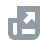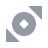Pay with
Markets
NFT
New
English
USDAccount FunctionsTutorialBinance Fan TokenBinance EarnCrypto Deposit/WithdrawalSpot & Margin TradingCrypto DerivativesFinanceAPISecurityBinance ConvertNFTVIP

# Binance Spot Grid Trading Parameters

2022-05-09 07:37

## What is Spot Grid Trading?

Spot Grid Trading is a quantitative trading strategy that uses trading bots to automate the buying and selling of assets on the spot market. It places orders in the market at preset intervals within a configured price range. When orders are placed above and below a set price, they create a grid of orders at incrementally increasing and decreasing prices. In this way, it constructs a Trading Grid.
You can use Binance Spot Grid Trading in volatile markets when prices fluctuate within a specific range. Grid Trading attempts to make profits on small price changes. Through quantitative trading, it can help you trade rationally and avoid FOMO where possible.

## Spot Grid Trading Parameters

1. Current Balance
Current Balance refers to the total balance of the Base and Quote Assets in your running Grid Orders.
Total Quote Asset Balance = Total Open Buy Order Price*Qty Per Order (represented in the Quote Asset)
Total Base Asset Balance = Total Number of Sell Orders*Qty Per Order (represented in the Base Asset)
In this example, the Last Price is 0.7760 USDT, the Qty Per Order is 14 XRP, and the Total Number of Sell Orders is 26.
Therefore, the Total Open Buy Order Price = 0.7696 + 0.7643 + 0.7590 + 0.7537 + 0.7484 = 3.795 USDT.
Total Quote Asset Balance = 3.795*14 = 53.13 USDT
Total Base Asset Balance = 26*14 = 364 XRP
2. Unrealized PnL
Unrealized PnL = Total Open Buy Order Price * Qty Per Order + Total Number of Sell Orders * Qty Per Order * Last Price + Reserved Fees in the Base Assets * Last Price + Reserved Fees in the Quote Assets - Total Investment
Last Price:
When the Grid Order is still running, the Last Price is the latest Market Price of the spot trading pair. If the Grid Order has ended, the Last Price is the Market Price at which the Grid Order ends.
Using the same example:
Total Investment: 369.6556 USDT
Last Price: 0.7760 USDT
Qty Per Order: 14 XRP
Reserved Fees: 6.0000 USDT + 15 XRP
Total Open Buy Order Price = 0.7696 + 0.7643 + 0.7590 + 0.7537 + 0.7484 = 3.795 USDT
Unrealized PnL = 3.795*14 + 26*14 * 0.7760 + 15 * 0.7760 + 6.0000 - 369.6556 = -16.4216 USDT
3. Matched Orders
A Matched Order refers to a Buy Order filled at the lower price and a Sell Order filled at the upper price.
The number of Matched Orders is used to calculate the Grid Profit.
In this example, Area 2 contains 1 Buy Order and 1 Sell Order. This is considered a Matched Order. In Area 1, however, only a Sell Order has been filled. Therefore, this trade isn’t a Matched Order. When the Buy Order is matched with a Sell Order in Area 1, the Buy Order would appear in Area 1 and the number of Matched Orders would increase by 1.
There are 178 total Matched Orders, which means this Grid Trading strategy has executed at least 178*2 = 356 orders.
4. Grid Profit
Grid Profit is the total profit generated by filled Buy and Sell Order pairs. It is displayed in the Quote Asset.
Profit of a Matched Order = Total Filled Sell Order Price - Total Filled Buy Order Price - Sell Order Trading Fees - Buy Order Trading Fees*Last Price
Last Price:
When the Grid Order is still running, the Last Price is the spot trading pair’s latest Market Price. If the Grid Order has ended, the Last Price is the price at which the Grid Order ends.
Note: If you use BNB to pay for trading fees, the BNB fees will be converted to Quote Assets at the Market Price when your Grid Order is running. If your Grid Order has ended, it will use the BNB price at the time the Grid Order ends to convert.
Take the above as an example. The Grid Order has ended, and the Stop Price is 46,617.70 USDT.
The profit of this Matched Order = 19.09794350 - 18.97818660 - 0.01336856 - 0.00000029*46,617.70 = 0.09286920 USDT
The Grid Profit is the sum of each Matched Order’s profit.
5. Total Profit
Total Profit = Grid Profit + Unrealized PnL
6. Annualized Yield
Annualized Yield = Total Profit / Total Investment*365 (days)*24 (hours)*60 (minutes) / Order Duration (minutes)
For example, the Total Profit is 31.30 USDT, the Total Investment is 688.04 USDT, and the Order Duration is 10 days 23 hours 55 minutes.
The Annualized Yield = 31.30 / 688.04*365*24*60 / (10*24*60 + 23*60 + 55) = 150.99%
7. Balance at Stop
Balance at Stop refers to the Quote and Base Assets in pending Grid Orders when the Grid Order ends. It uses the same calculation as the current balance.
8. PnL Asset
This means all PnL of your Grid Orders will be converted to the Quote Asset and will be calculated based on the Quote Asset’s value.
For example, when you trade BNB/BUSD, your PnL will be shown in BUSD.
9. Profit per Grid (Profit/Grid)
Profit/Grid is the profit percentage after matching the Buy and Sell Orders on each Grid. Depending on the trading mode (Arithmetic or Geometric), you can calculate the Profit/Grid by the Grid’s Upper Limit, the Grid’s Lower Limit, the number of Grids, and the trading fees (c): 0.1%.
The Profit/Grid for Arithmetic Grids is calculated as a range. The minimum Profit/Grid is generated by the lowest Grid, and the maximum Profit/Grid is generated by the highest Grid.
Price Difference (d) = (Grid Upper Limit - Grid Lower Limit) / Number of Grids
The Maximum Profit/Grid = (1 - c)*d / Grid Lower Limit - 2c
The Minimum Profit/Grid = (Grid Upper Limit*(1 - c)) / (Grid Lower Limit - d) - 1 - c
The Profit/Grid for Geometric Grids is a fixed value, since the price ratio of each Grid price is the same.
Price Ratio (r) = (Grid Upper Limit / Grid Lower Limit) ^ (1 / Number of Grids）
The Profit/Grid = (1 - c)*r - 1 - c
For example, the Grid Upper Limit is 450 and the Lower Limit is 400. The number of Grid is 5, and the trading fee (c) is 0.1%.
If the Grid is Arithmetic, the Price Difference (d) = (450 - 400) / 5 = 10
Maximum Profit/Grid = (1 - 0.1%)*10/400 - 2*0.1% = 2.29%
Minimum Profit/Grid = (450*(1 - 0.1%)) / (450 - 10) - 1 - 0.1% = 2.07%
If the Grid is Geometric, then the Profit/Grid = (1-0.1%)*(450 / 400) ^ (1 / 5) - 1- 0.1% = 2.18%
10. Qty Per Order
Qty Per Order refers to the quantity of each order at a different price during Grid Trading. The Qty Per Order is only confirmed after the Grid Order is successfully created. The system will automatically calculate the Base Asset needed to be reserved for the trading fees and the Quote Asset needed to place Buy Orders according to the investment amount entered.
After calculating all the assets needed, the maximum quantity that could guarantee the success of each Grid Order is the Qty Per Order.